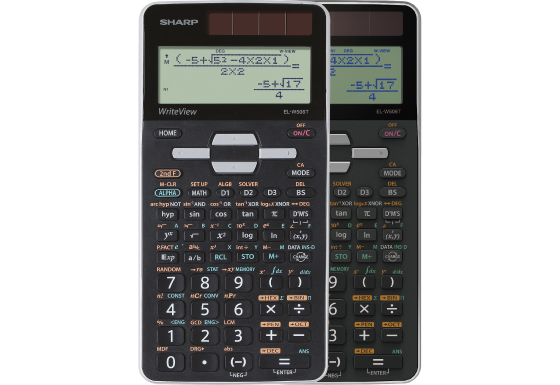## EL-W506T

Calculator EL-W506T with large dot matrix "WriteView" display, which allows you to write and display expressions as on paper, is the most advanced scientific calculator in the Sharp portfolio. It contains 640 statistical, trigonometric and mathematical functions including metric conversions, physical constants and probability distributions.Specification

• Built-In Functions: 640
• Entry System: Algebraic (D.A.L.)
• Power Source: Solar & Battery
• Dimensions and Weight: 166 × 80 × 15 mm, 108 g

Mathematical Functions

• Fractions
• Equation Solver (Newton)
• System of Linear Equations
• Matrix and Vector Calculations
• Numeric Derivative and Integral
• Complex Number Calculation
• Training (DRILL) Function

Statistics

• 1-Variable Statistics
• 2-Variable Statistics
• Regression Calculations: 7
• Mean, Standard Deviation
• nCr, nPr, n!
• Random Numbers

Scientific Functions

• Metric Conversions
• Physical Constants

Memory

• Multi-Line Playback
• Memory Variables: 9

Notation and Decimal Places

• FSE Display (SCI, FIX, ENG)

Trigonometric Functions